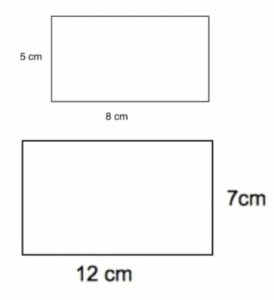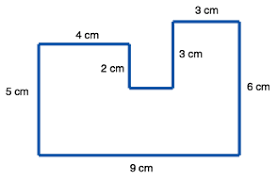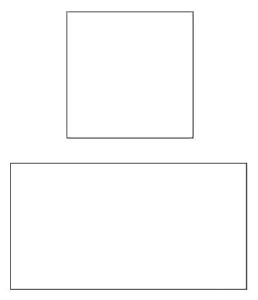# Perimeter of Regular and Irregular Shapes (Primary 6)

MATHEMATICS

SECOND TERM

WEEK 4/PRIMARY 6

WEEK/PRIMARY 5

THEME – MEASUREMENTS

PREVIOUS LESSON – Second Term Scheme of Work and Plan Lesson Notes for MATHEMATICS Week 1 to Week 12 Primary Schools

TOPIC – PERIMETER OF REGULAR AND IRREGULAR SHAPES

PERFORMANCE OBJECTIVES

By the end of the lesson, the pupils should have attained the following objectives (cognitive, affective and psychomotor) and should be able to –

discover that different rectangle with the same area have different perimeters.

ENTRY BEHAVIOUR

INSTRUCTIONAL MATERIALS

The teacher will teach the lesson with the aid of cardboard showing rectangle of equal rectangle areas but different perimeters.

METHOD OF TEACHING – Choose a suitable and appropriate methods for the lessons.

Note – Irrespective of choosing methods of teaching, always introduce an activities that will arouse pupil’s interest or lead them to the lessons.

REFERENCE MATERIALS

1. Scheme of Work

2. 9 – Years Basic Education Curriculum

3. Course Book

4. All Relevant Material

5. Online Information

CONTENT OF THE LESSON

LESSON ONE – INTRODUCTION TO RECTANGLES

Pupil’s Activities 1 – Properties of Cuboid

Teacher’s Activities – Questions

1. The objects below is called ______________.2. What shape does represent in mathematics?

3. How many faces does the objects has?

4. Many corners or vertices does it have?

5. The object is used for ______________.

6. Mention 5 objects that can be packaged inside.

Note – Guides or leads the pupils where necessary.

Pupil’s Response

1. Carton or box

2. Cuboid

3. Six (6) faces

4. Eight vertices (corners)

5. Packaging

6. Indomie, wine, milk, television, radio, Maclean, chalk, marker, etc.

Teacher’s/Pupil’s Activities 2 – Introduction to Rectangle

Teacher’s Activities – Cut one of the faces of the carton. Asks pupils to draw and name it.Pupil’s ResponseRectangle

Pupil’s Activities 3 – Lengths of Rectangle

Instructions/Questions

1. Measure each length of the rectangle.

2. Compare each length with another.

3. Use measurements to describe what rectangle means.

4. Mention 5 objects with rectangular face or shape.

Pupil’s Response

1. 10 cm, 7 cm, 10 cm and 7cm.

2. Two of the lengths are equal to each other.

3. Rectangle is a shape with 4 length. Two of its lengths are equal to another.

4. Board, sheet of paper, door, wall or floor, top of desk, etc.

Teacher’s remark – A rectangle is a 4 sided with opposite parallel lengths are eual length. It is one of the group called quadrilateral.

LESSON TWO – PERIMETER OF A RECTANGLE

Pupil’s Activities 1 – Measurement of Rectangles

Draw two (2) rectangles with 7 cm by 3 cmPupil’s Activities 2 – Perimeter of a Rectangle

Add the lengths of the rectangle drawn.

7 cm + 3 cm + 7 cm + 3 cm

= 20 cm.

Teacher’s remark – The sum of the lengths of a rectangle is called perimeter of a rectangle. 7 cm + 7 cm + 3 cm + 3 cm = 20 cm. What is perimeter?

Teacher’s/Pupil’s Activities 2 – Perimeter of A Rectangle

Find the perimeter of the rectangle.Perimeter (P) of a rectangle,

= a + b + a + b

= a + a + b + b

= 2a + 2b

= 2 (a + b)

Pupil’s Activities 3 – Exercises/Assignment

1. Find the perimeter of1. Draw a rectangle of 15 cm by 8 cm and 8 by 10 cm.

LESSON THREE – PERIMETER IRREGULAR SHAPES

Pupil’s Activities 1 – Regular and Irregular Shapes

Examine the shape and compare it with a rectangle.Teacher’s remark – Listen to the pupils and discuss the number of rectangle in the irregular shape.

Teacher’s/Pupil’s Activities 2 – Perimeter of Irregular Shapes

1. How many lengths does the below shape has?2. Name each length A to F.

3. What is D and E?

Guide pupils in this activities.

Pupil’s Response,

A = 12, B = 10, C = 7, D = 10 – 2, E = 12 – 7 and F = 2

Perimeter (p)

= 12 + 10 + 7 + (10 – 2) + (12 – 7) + 2

= 12 + 10 + 7 + 8 + 5 + 2

= 44.

Pupil’s Activities 3 = Exercises/Take Home

Find the perimeterLESSON FOUR – PERIMETER OF A SQUARE

Pupil’s Activities 1 – Measurement of Square

Measure the lengths of the square and rectangles. What is the different between their lengths?Pupil’s comment – The square has equal lengths. While the rectangle has the opposite lengths equal.

Pupil’s Activities 2 – Perimeter of a Square

Teacher’s comment – Perimeter is the sum of the lengths of a plane shapes.

Find the perimeter of square in activities 1.

Pupil’s Activities 3 – Perimeter of a square (4L)

Perimeter (P)

= L + L + L + L

= 4L

Pupil’s Activities 4 – Exercises/Take Home

LESSON FIVE – RECTANGLES OF THE SAME AREA HAVING DIFFERENT PERIMETERS

Pupil’s Activities 1 – Rectangles with the same area and different perimeters.

Measure and find the areas of the following rectangles,Rectangle A

Length – 8 cm

= 8 cm x 5 cm

= 40 cm²

Rectangle B

Length – 10 cm

Area = 10 cm x 4 cm

= 40 cm²

Pupil’s Activities 2 – Find the perimeter of the rectangles in activities 1

Rectangles A

Perimeter = 2(8 cm + 5 cm)

= 2 x 13 cm

= 26 cm.

Rectangle B

Perimeter = 2 (10 cm + 4 cm)

= 2 x 14 cm

= 28 cm.

Teacher’s remark – The two rectangles have the same area (40 cm2) but have different perimeters. Their perimeters are 26 cm and 28 cm respectively.

Pupil’s Activities – Exercises/Take Home

Copy and complete the following table and obtain different rectangles with the same area.PRESENTATION

To deliver the lesson, the teacher adopts the following steps:

1. To introduce the lesson, the teacher revises the previous lesson. Based on this, he/she asks the pupils some questions;

2. Guides pupils to draw two rectangles of dimensions 7 cm by 3 cm and 1o cm by 15cm.

Pupil’s Activities – Find the perimeters of the two rectangles.

3. Guides pupils to prove that they have equal area but different perimeters.

Pupil’s Activities – Compare their results.

CONCLUSION

• To conclude the lesson for the week, the teacher revises the entire lesson and links it to the following week’s lesson.

LESSON EVALUATION

Pupils to calculate perimeters of rectangles with equal areas but with different lengths and breadths.